# KMP 算法

KMP（Knuth-Morris-Pratt）算法在字符串查找中是很高效的一种算法，假设文本字符串长度为n，模式字符串长度为m，则时间复杂度为O(m+n)，最坏情况下能提供线性时间运行时间保证。

《算法导论》和其他地方在讲解KMP算法的时候，过于数学化且晦涩难懂，我也因此迷惑了很长时间。后来看《算法（第四版）》部分的讲解，对其中最复杂的Next数组有了重新的认识。我这里也希望用通俗的语言来将我的理解分享出来。

KMP算法的本质是构造一个DFA确定性有限状态自动机），然后通过自动机对输入的字符串进行处理，每接收一个字符，就能转移到一个新的状态，如果自动机能够达到特定的状态，那么就能够匹配字符串，否则就匹配失败。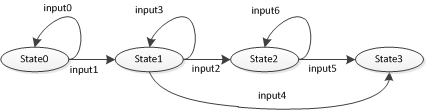public static int search(String pat,String txt){
int j,M = pat.length();
int i,N = txt.length();
for (i = 0, j = 0; i < N && j < M; i++){
if (txt.charAt(i) == pat.charAt(j)){
j++;
}
else{
i -= j;//go back
j = 0;
}
}
if (j == M){
return i - M;//match
}
else {
return N;//not match
}
}

public static int search(String pat,String txt){
int j,M = pat.length();
int i,N = txt.length();
for (i = 0, j = 0; i < N && j < M; i++){
if (txt.charAt(i) == pat.charAt(j)){
j++;
}
else{
j = dfa[txt.charAt(i)][j];//use an array dfa[][]
}
}
if (j == M){
return i - M;//match
}
else {
return N;//not match
}
}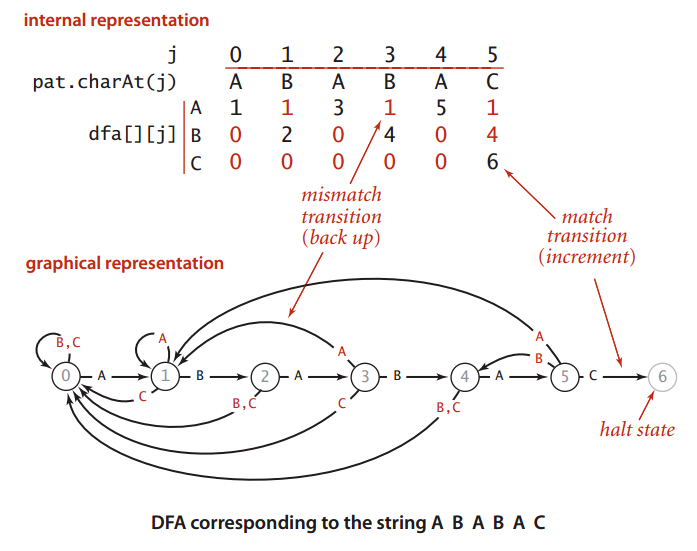dfa[][]数组对的每一列都对应一个状态，每一行都对应一种输入。

dfa[pat.charAt(0)] = 1;
for (int X = 0, j = 1; j < M; j++)
{
// Compute dfa[][j].
for (int c = 0; c < R; c++)
dfa[c][j] = dfa[c][X];
dfa[pat.charAt(j)][j] = j+1;

X = dfa[pat.charAt(j)][X];
}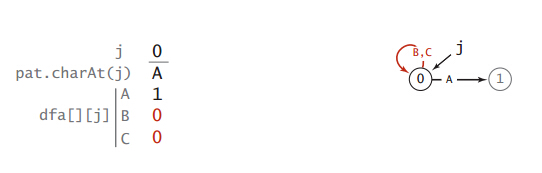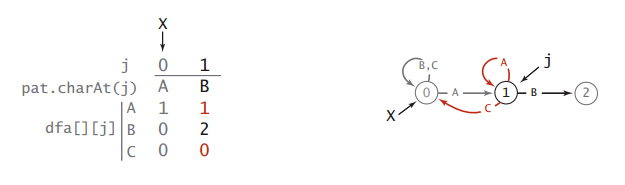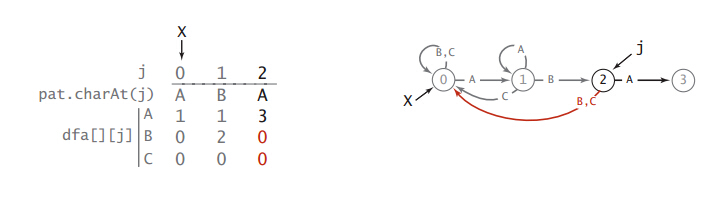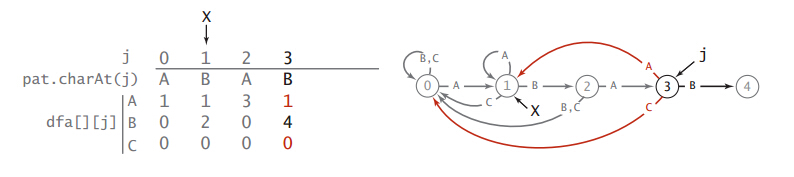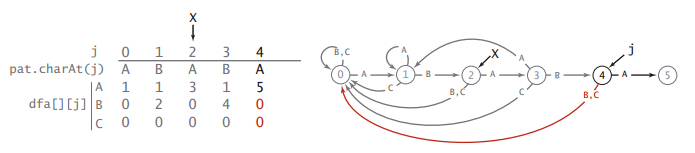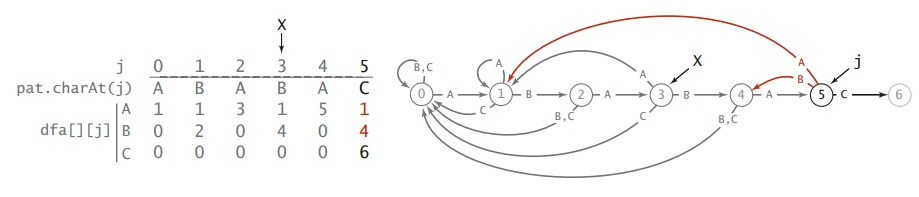X = dfa[pat.charAt(j)][X];

《算法（第四版）》最后提到：

• 《大话数据结构》
• 《算法导论》
• 《算法（第四版）》
12-2605-022623
04-159726
04-254万+
02-28692
03-2225万+
03-162万+
02-0726万+
11-134639
©️2020 CSDN 皮肤主题: 大白 设计师:CSDN官方博客